No doubt we’re all familiar with circles: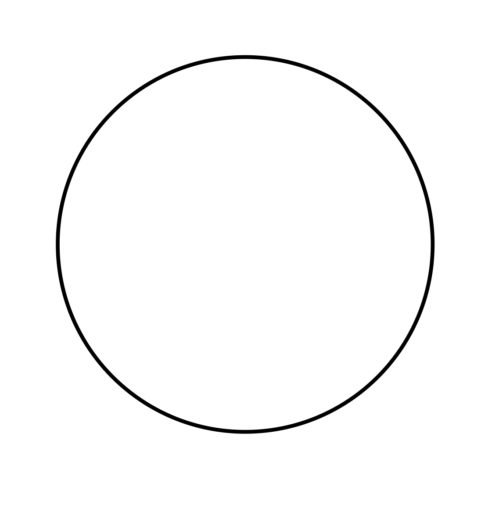Less familiar, though, may be the many technical terms for certain parts of the circle — the kinds of terms that most people leave behind in high school. But knowing what those terms mean can help you on the GRE by giving you a vocabulary that helps you to understand the formulas and the problems (it’s easier to learn how to find the area of a circle’s sector, rather than “the bit of the circle left over when you cut off the circular bit”).

Circle: A 2D shape whose points are always the same distance from some central point.

Center: The center of a circle is the point within the circle from which every point of the circle is the same distance. We often label the center and use it to identify the circle (e.g. “the circle whose center is at A”).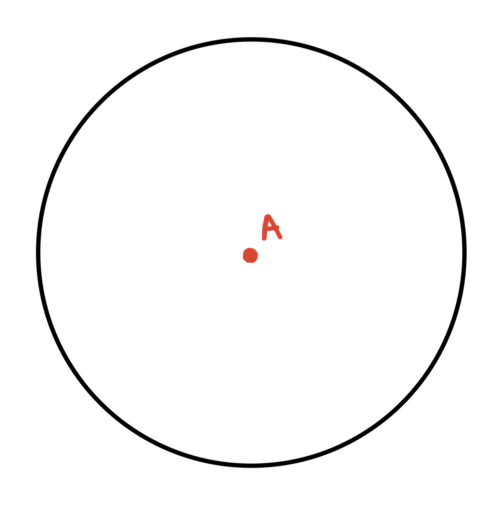Radius: Any line from the center of a circle to any point on the circle. We often use the variablefor the length of the radius.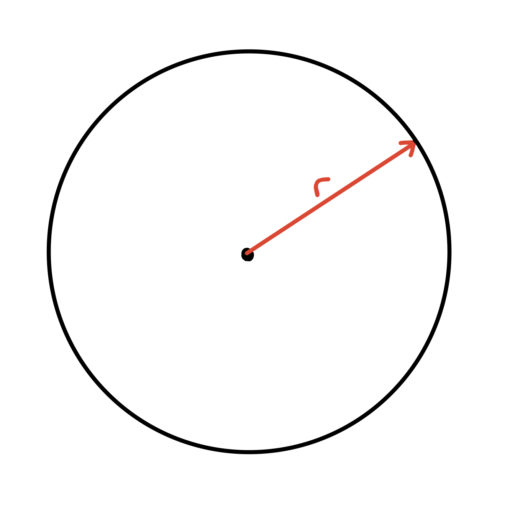The radius of a circle is often very important because it allows us to find the area of that circle:

Area of a Circle: The area of a circle with radiusisDiameter: Any line that connects two points on the circle and the center of the circle. We often use the variablefor the length of the diameter.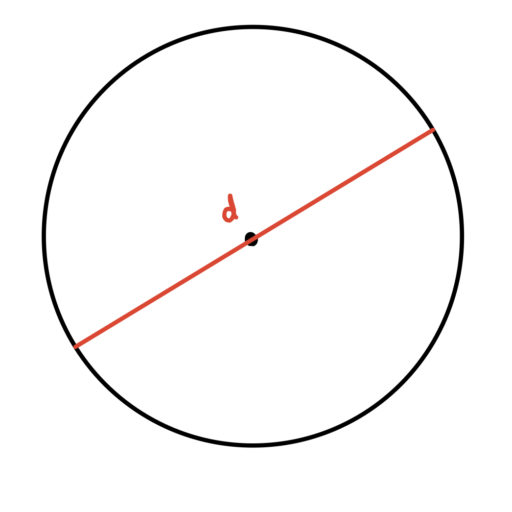And of course, using the diameter, we can always find the radius of a circle:

Diameter Formula: For a circle with radius, the diameterCircumference: The distance around the circle.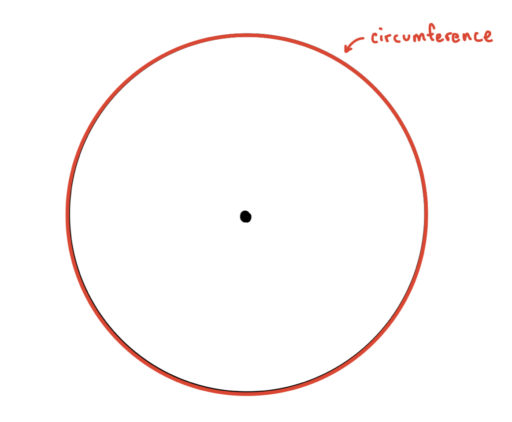And we can find the circumference of a circle using the diameter:

Circumference Formula: The circumference of a circle with a radiusisThus, the area of a circle, its radius, its diameter, and its circumference are all related. Using any one of these values, we can find the other three!

Chord: Any line segment that connects two points on a circle.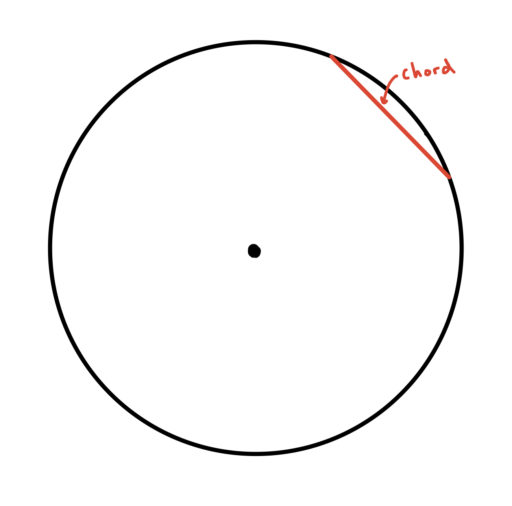Note also that:

Diameter is Longest Chord: The diameter of a circle is also a chord of the circle and, in fact, it is the longest chord on a circle.

Arc: Any portion of a circle’s circumference located between two points on the circle.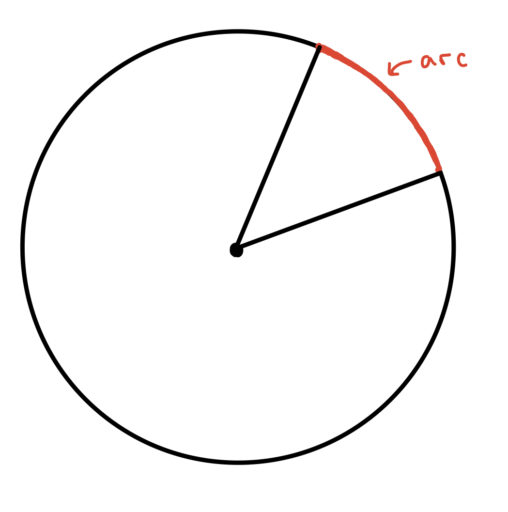Sector: A portion of the circle enclosed by two radii and an arc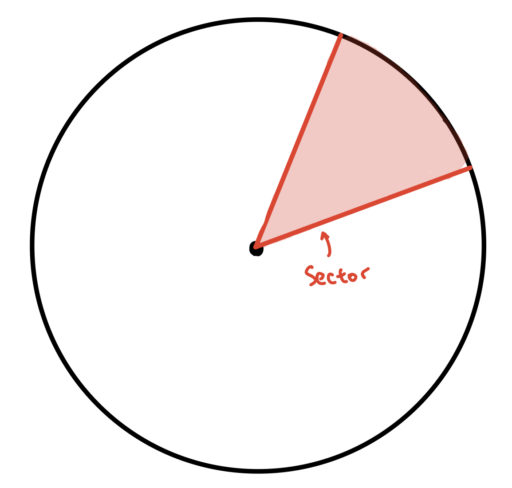Some GRE problems will ask you to find the area of some sector of the circle. We will talk about how to handle those problems later (%TODO insert hyperlink here).

Tangent: A straight line that touches a curve or circle at a single point

Point of Tangency: The point at which a tangent line touches a curve. Note that the tangent line (in red below) will be perpendicular to any line connecting the center and the point of tangency.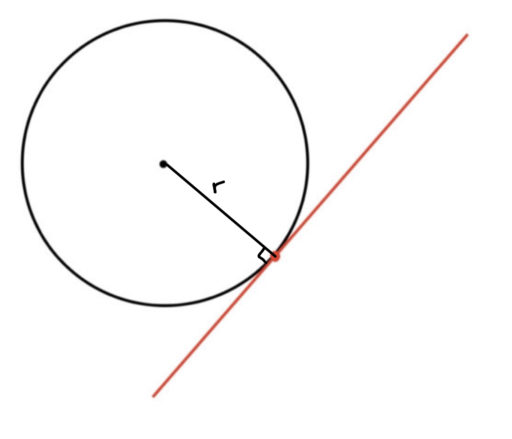Finally, circles can be drawn within or around other shapes:

Inscribed: Circles can be inscribed within other shapes. An inscribed circle is the largest possible circle that can be drawn wholly within another shape. For example: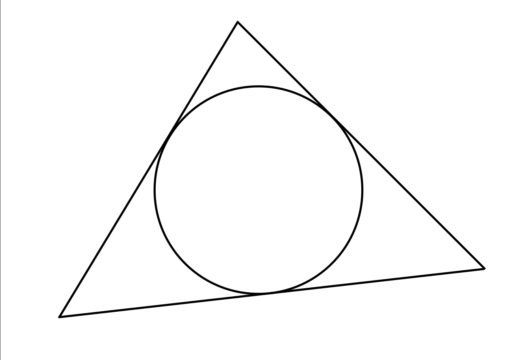Circumscribed: Circles can also be drawn around other shapes. A circumscribed circle is the smallest possible circle that can be drawn wholly outside of another shape. For example: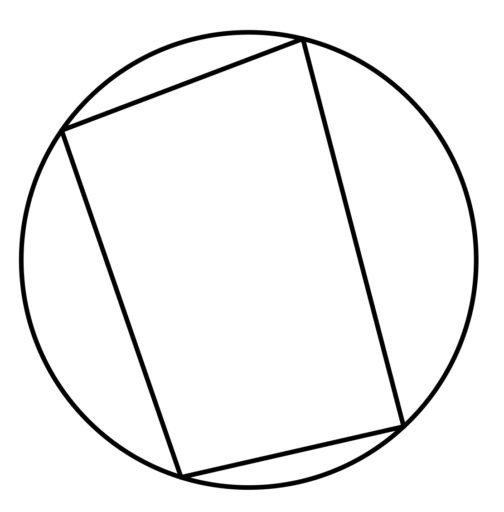And circles can share a center:

Concentric Circles: Two circles are concentric if they share the same center.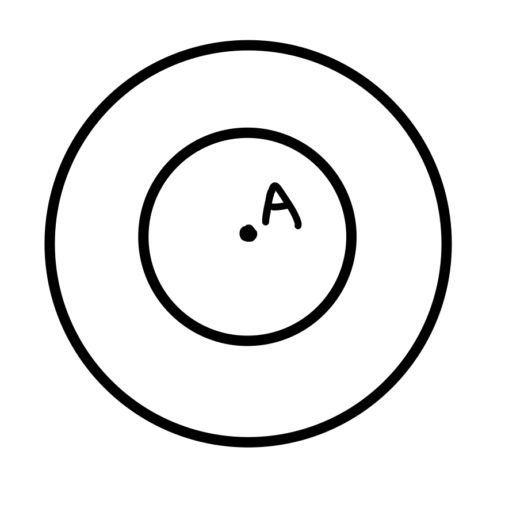Practice Problems:

1. Draw a circle inscribed within a square

3. The radius of the below circle is 1. What is the area of the shaded region?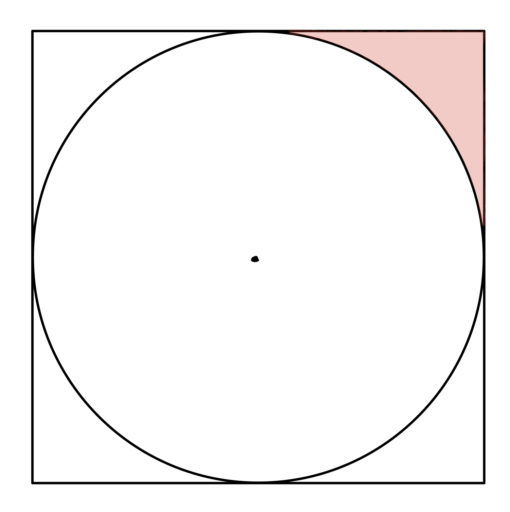4. Which is longer?A.is longer.
B.is longer.
C. They are equally long.
D. It cannot be determined.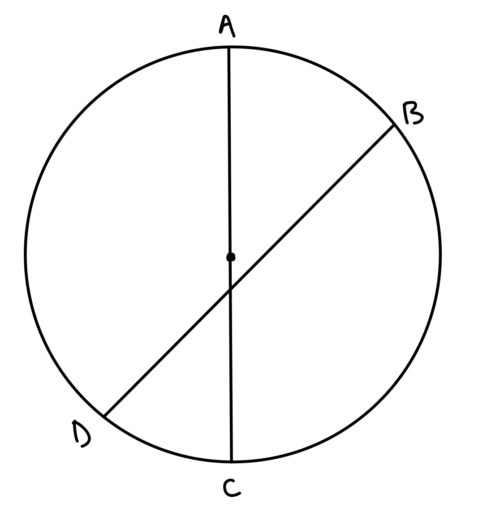5. The radius of the following inscribed circle is 5, and the radius drawn connects to a point of tangency. What is the area of the shaded region?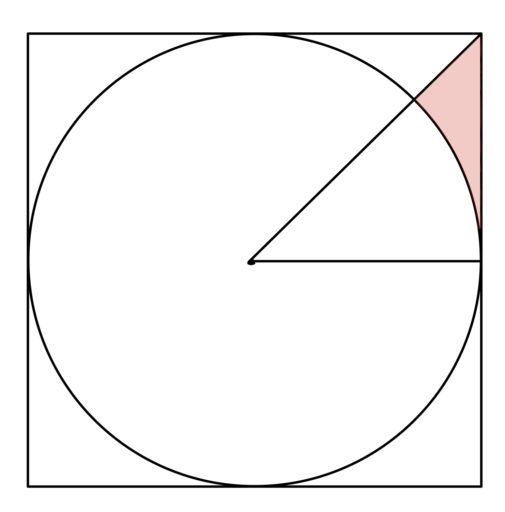6. The shaded region has an area of 5. What is the area of the circle?